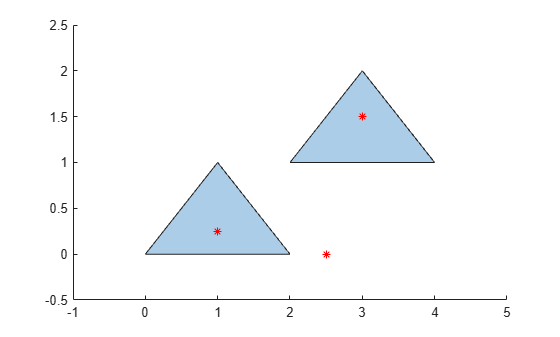isinterior

Query points inside polyshape

Description

example

TFin = isinterior(polyin,x,y) returns a logical vector whose elements are 1 (true) when the corresponding x-coordinates and y-coordinates in the vectors x and y are in a polyshape. A point is in a polyshape if it is either in a solid region or on one of the boundaries.

TFin = isinterior(polyin,P) represents the query points in a 2-column matrix P. The first column of P contains the x-coordinates of the query points, and the second column contains the corresponding y-coordinates.

[TFin,TFon] = isinterior(___) returns an additional logical vector for either of the previous syntaxes. The elements of TFon are 1 when the corresponding query points are on a boundary of polyin.

Examples

collapse all

Create a polygon containing two solid regions.

x1 = [0 1 2];
y1 = [0 1 0];
x2 = [2 3 4];
y2 = [1 2 1];
polyin = polyshape({x1,x2},{y1,y2});
plot(polyin)
hold onDefine three points, and determine if they are in the polygon.

x = [1 2.5 3];
y = [0.25 0 1.5];
TFin = isinterior(polyin,x,y)
TFin = 3x1 logical array

1
0
1

plot(x,y,'r*')Input Arguments

collapse all

Input polyshape, specified as a scalar.

Data Types: polyshape

x-coordinates of query points, specified as a scalar or vector.

Data Types: double | single

y-coordinates of query points, specified as a scalar or vector.

Data Types: double | single

2-D vertices, specified as a 2-column matrix. The first column of P contains the x-coordinates of the query points, and the second column contains the y-coordinates.

Data Types: double | single

Output Arguments

collapse all

Interior point indicator, returned as a scalar or vector. An element of TFin is 1 when the corresponding query point is either in a solid region of the input polyshape or on a boundary.

Data Types: logical

Boundary point indicator, returned as a scalar or vector. An element of TFon is 1 when the corresponding query point is strictly on a boundary of the polyshape.

Data Types: logical